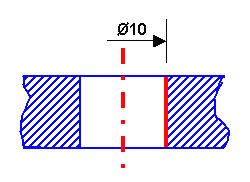# Creating Half-DimensionsThis task will show you how to create a half-dimension. You can create half-dimensions on distance, angle, diameter cylinders, radius cylinders made out of two selections, diameter edges and diameter tangents. You cannot create half-dimensions on cumulated dimensions.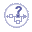Half-dimensions are useful in the case of revolved features or elements using a plane symmetry, as they let you create the dimensions only on half the geometry.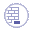Open the Brackets_views05.CATDrawing document.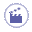1. Click Dimensions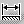in the Dimensioning toolbar.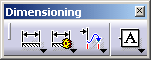2. Click a first element in the view. For example, an edge.

3. If needed, click a second element in the view. For example, another edge.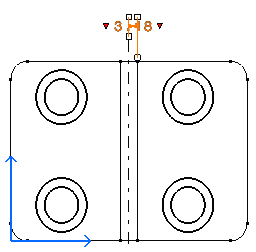4. Right-click the dimension and select Half Dimension.

The half-dimension appears. Only one extension line is displayed. The dimension line is shortened with specific overrun, gap and length. The value is not centered on the dimension line. The attributes mentioned in Dimension parameters drive the dimension graphic display.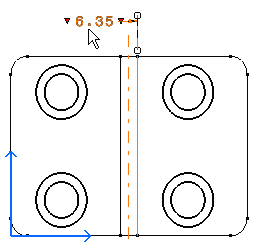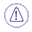• Once you select the half-dimension option from the contextual menu, all the following dimensions you create will be assigned the half-dimension mode. If you want to create dimensions in the standard mode, go back to the contextual menu and de-activate the Half Dimension option.
• You can create a half-dimension directly by selecting first an axis line and then an other element (which is not an axis). The half-dimension value will be the double of the measured value between the elements. If you don't want a half-dimension to be created when selecting such elements, uncheck Half Dimensions from the contextual menu (right-click) when creating the dimension.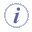• The dimension value is doubled when they are made out of two selections (distance, angle, 2D diameter cylinder, radius cylinder) but not for dimensions made out of one selection (angle on cone, 3D diameter cylinder, diameter edge, diameter tangent).
• Associativity in the case of half-dimensions is different from associativity in the case of standard dimensions. For example, the half distance dimension below is associated to the axis and the element, whereas a standard dimension is associated to both symmetrical elements.
 Standard distance dimension: Half distance dimension: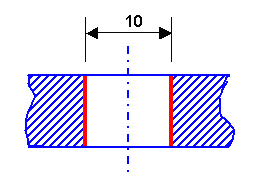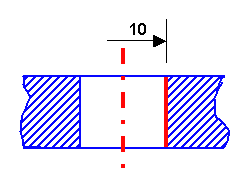Diameter and radius dimensions are usually created with one selection in 3D. If the dimension is created with two selections, for instance an edge coming from a 3D revolution and another element, the dimension will be not associative. To create the dimension below, you must select only the left or the right side of the cylinder and then right-click on the dimension and select Half Dimension.

 Half diameter dimension: